555 Timer

According to Wikipedia and other sources, the 555 timer is the most popular integrated circuit ever manufactured, with billions having been sold. Frequency and duty cycle are determined by the values of an attached capacitor and resistors, as explained in the TTL Cookbook and elsewhere. The following calculators estimate either the frequency that will be generated from given capacitor and resistor values, or the approximate capacitance needed to achieve a target frequency, given specified test resistor values.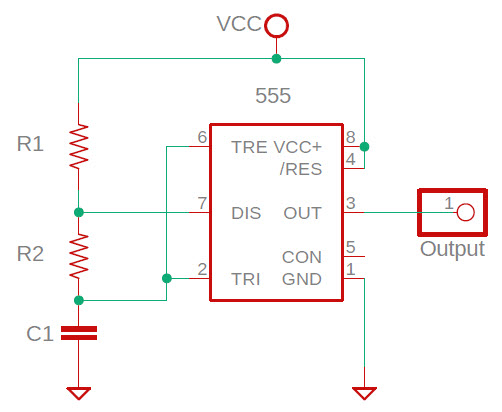555 Capacitance Estimator

Note: Observe 555 minimum and maximum R/C values (TTL Cookbook, pages 172-173.)

Coax velocity factor or length

There are several ways to determine the velocity factor of a known length of coax, or conversely the length given the velocity factor. Two of these methods are illustrated below. The first is direct measurement of the round-trip reflection time for a signal in an unterminated length of coax. The test setup is simple. A square-wave function generator or oscillator is connected to an oscilloscope. I use a BNC ‘T’ connector at the oscilloscope input jack. The ‘unknown’ coax is connected to the other side of the T jack. Frequency is not critical.  Make the time scale of the oscilloscope (horizontal axis) sufficiently sensitive to read reflection time to within a few nanoseconds. For a 50 foot length of coax, 25 nS per scale division should work.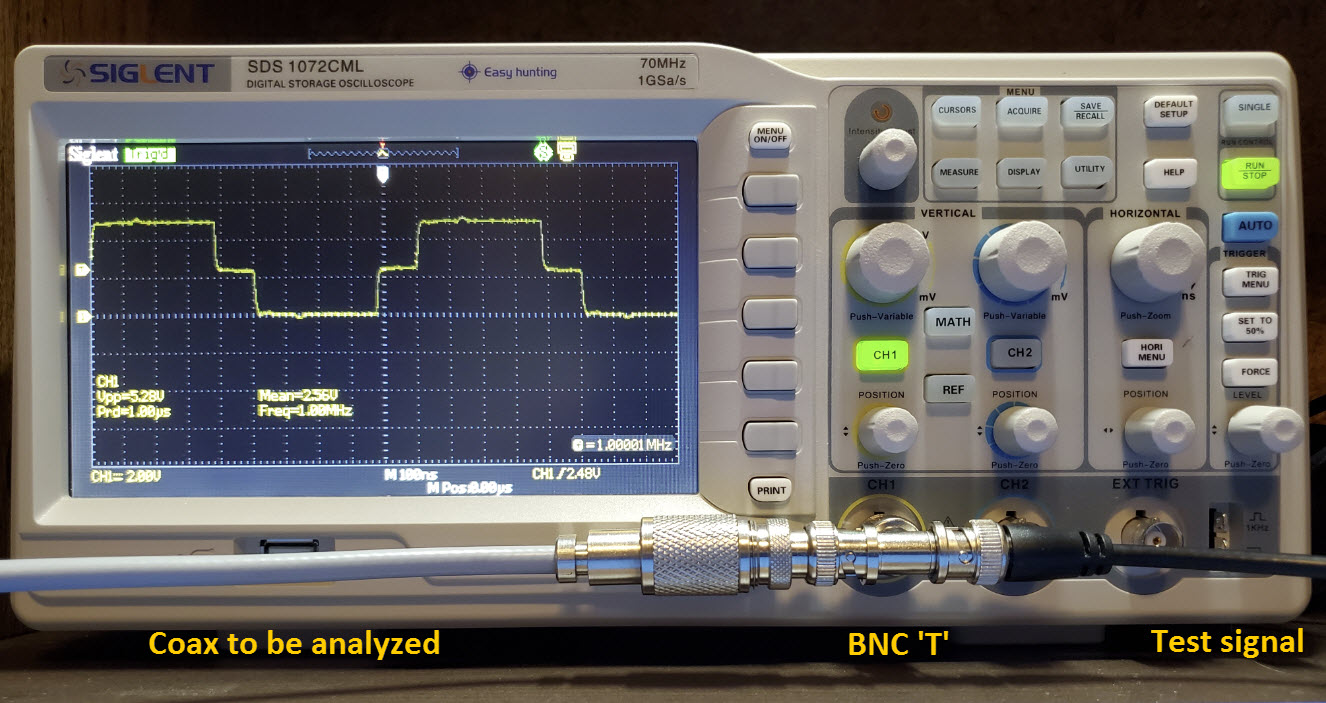Resonant frequency method

An antenna analyzer can be used to ascertain the resonant frequency of a length of coax, e.g. at 1/4, 1/2, etc. wavelengths. Resonant frequency can then be used in a way similar to reflection time, to estimate length given velocity factor, or conversely to determine velocity factor, given length. The test setup is shown in the following illustration. (The illustrated resonance is at 1/4 wavelength.)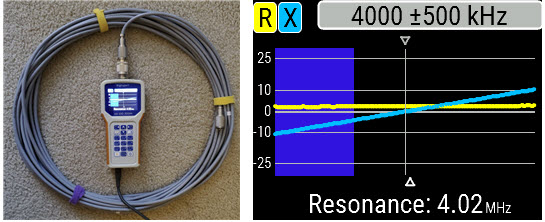Length

Select the appropriate wavelength fraction for the resonance (default is 1/4λ), or enter a value in the input box if none of the selectable fractions apply.

Inductance from half-voltage frequency

I had ordered an electronics kit and sorted the components in preparation for assembling the kit. There was one wrong valued component and one that I wasn't sure about. The questionable component was an inductor and, while it is simple to test an unknown resistor with a multi-meter, to measure inductance an LCR meter (which I did not have at the time) would be needed. Google to the rescue! The page at http://www.dos4ever.com/inductor/inductor.html presents a simple method to measure an unknown inductance (from a few to a few hundred μH). Once the procedure described in this resource is carried out, the mathematical calculation is even simpler, and can be performed on an ordinary calculator. Even so, why not code the calculation so that inductors can be measured quickly, with minimal effort.

Test example: Assume the inductor has negligible resistance and that upon carrying out the procedure described in the above-referenced page, the frequency that resulted in a half-amplitude reading was 1,175,000 Hz (enter values in Hz, not KHz or MHz). Entering this value in the frequency box below without commas (leave the resistance box empty) results in an inductance measure of 3.9 μH, which happens to be the value that the inductor in question should have had.

If the inductor has a significant internal resistance (can be measured with an ohmmeter) then enter this value in the resistance box before clicking ‘compute.’

Note: The experimental method is fully described at the page linked in the introductory paragraph above.

Permeability of a ferrite rod

A 5-pack of ferrite rods of unspecified type or mix were advertised as suitable for [making] a radio antenna. I thought it should be possible to ascertain some properties of these rods by first measuring inductance of a test coil, then using this measured value together with other parameters to compute the material’s permeability: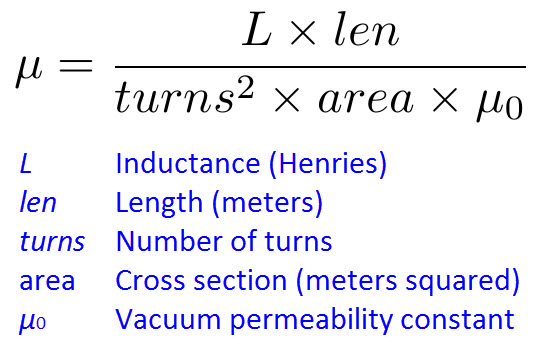Magnetic permeability (μ)

Select units for length and diameter.

Select units for inductance.

μ postscript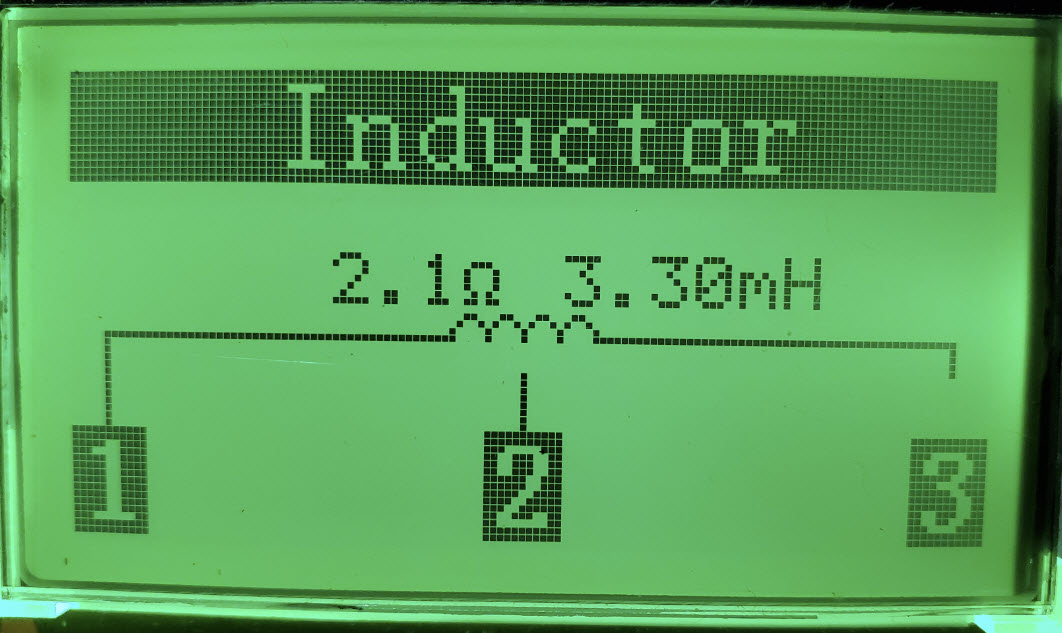I am not confident about this calculation. It did not resolve the type of rods in the 5-pack I’d bought. These rods are each 140 mm in length. In cross-section they are not exactly round. (See photo - Are they flattened for gluing?) Outer diameter (neglecting flattening) is 10 mm and the width between shaved edges is approximately 8.5 mm. For the μ calculation (which assumes roundness in this implementation) a middle value (9.25 mm) was entered for diameter. After winding a test coil (240 turns of enameled wire) I measured inductance in three different ways. The results were in approximate agreement, and for the calculation I used 3.3 mH. With these values as input, the calculator gives μ = 95.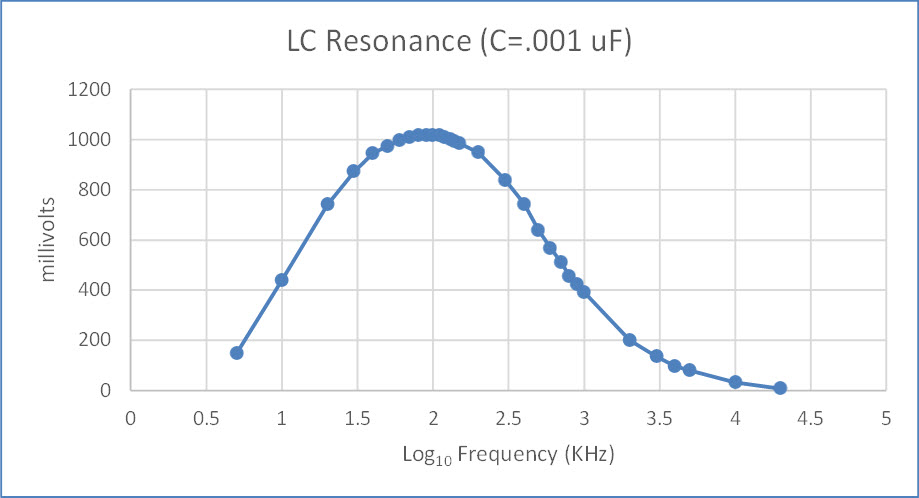Equal Payment Amount (Also known as Amortization)

You are given a principal loan amount (P), an interest rate (i), and a number of equal payments (N). Interest is expressed as a fraction or proportion per payment.  For example, if the loan is to be paid monthly and the interest is 6% per year, fractional interest would be 6÷1200 or .005. The divisor 1200 reflects conversion from percent to fraction (÷100) and from annual to monthly (÷12). The number of payments (N) refers to the total number over the course of the loan.  For example, if payments are monthly and the loan is for 6 years, N = 6×12 = 72. Let A stand for the equal payment amount: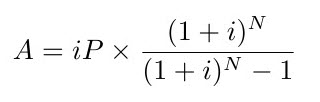Many years ago (i.e., before Internet and before personal computers) a calculator salesman asked me to program this problem for his machine, and promised a case of scotch whiskey as payment. I never saw a drop of scotch, but working out a program for the problem was reward enough. Back then it wasn’t JavaScript!

Example: Suppose you wish to borrow \$25,000.00 at an annual periodic rate of 6%, and make monthly payments for 6 years. Enter 25000 as a number into the first box (omit the dollar sign and commas), .005 in the second box (see first paragraph above), and 72 in the third box. Then click the ‘Compute Amount’ button. For these input values the monthly payment should be \$414.32. This amount consists purely of principal and interest. It does not include any add-on charges that may be included in a real loan, such as taxes, insurance, and so forth.

The author makes no claim as to the accuracy or completeness of information presented on this page. In no event will the author be liable for any damages, lost effort, inability to reproduce a claimed result, or anything else relating to a decision to use the calculators or supplemental information on this page.